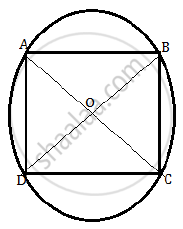# All the vertices of a rhombus lie on a circle. Find the area of the rhombus, if the area of the circle is 1256 cm2. (Use π = 3.14) - Mathematics

Sum

All the vertices of a rhombus lie on a circle. Find the area of the rhombus, if the area of the circle is 1256 cm2. (Use π = 3.14)

#### Solution

Given that the area of the circle is 1256 cm2.

pir^2 = 12563.14 xx r^2

3.14xxr^2 = 1256

r^2 = 1256/3.14

r^2 = 400

r = 20 cm

If all the vertices of a rhombus lie on a circle, then the rhombus is square.
Consider the following figure.Here A, B, C and D are four points on the circle.

Thus, OA = OB = OC = OD = radius of the circle.

⇒ AC and BD are the diameters of the circle.

By Pythagoras theorem, we have,

2AD^2 = (2 xx 20)^2  ......[AD = CD]

2AD^2 = (40)^2

AD^2 = 1600/2

AD^2 = 800 cm^2

If AD is the side of the square, then AD2 is the area of the square.

Thus area of the square is 800cm2

Concept: Circumference of a Circle
Is there an error in this question or solution?

#### APPEARS IN

NCERT Mathematics Exemplar Class 10
Chapter 11 Area Related To Circles
Exercise 11.4 | Q 12 | Page 134

Share